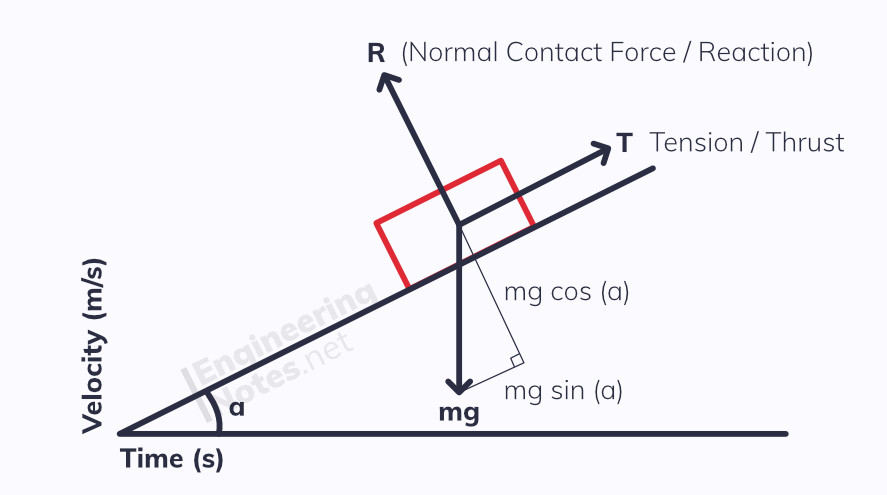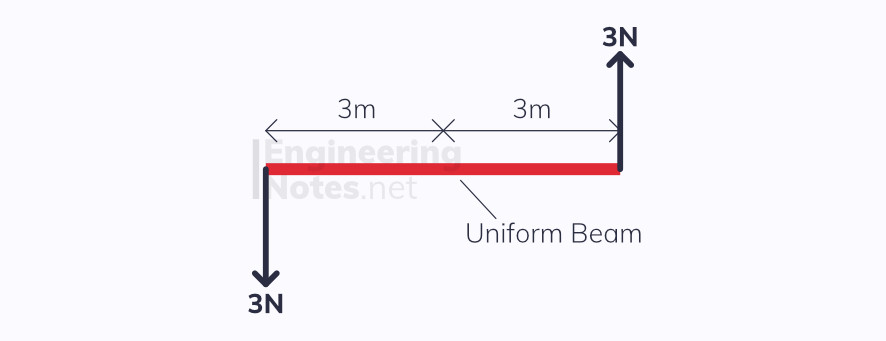#### Notes by Category University Engineering

Electronics*
Mathematics*
Mechanics & Stress Analysis*
Rate these notesNot a fanNot so goodGoodVery goodBrillRate these notes

# Forces & Newton's Laws

First off, it is important to distinguish between mass and weight. Mass is a physical property of an object, dependent on the amount of matter the object is made up of. The weight of an object is the gravitational force acting on its mass. On earth, where g = 9.81, the weight is 9.81 x the mass:

weight = mass x gravity W = mg = 9.81m

## Newton's Laws of Motion

### Newton's 1st Law:

An object will stay at a constant velocity, or motionless, unless it experiences a resultant force.

### Newton's 2nd Law:

The resultant force on an object is directly proportional to the rate of change of momentum of the object, and acts in the same direction.

Therefore, F = Δp / Δt, which is commonly written as F=ma

### Newton's 3rd Law:

For every action, there is an equal but opposite reaction (equal in magnitude and type of force).

## Representing Forces

Weight acts down from an object's centre of gravity. Other forces can act in any direction, and so to avoid confusion it is best to draw a force diagram:The length of arrow should represent the magnitude of the force. Single headed arrows represent forces.

If a system is in equilibrium - meaning there is no net resultant force and it is motionless or at constant velocity - then all the force arrows will make a closed shape. The example above would give a triangle.

So yeh, trigonometry is used a lot...

## Drag & Terminal Velocity

Though not modelled mathematically at A-Level, when objects fall through fluids (liquids & gasses) there is significant drag that slows them down. This means that unless in a vacuum, there is no such thing as free fall as acceleration is not constant.

The magnitude of the drag force depends largely on the shape and texture of the object, the density of the fluid, and the speed of the object - the greater the speed, the greater the drag force, until it equals the weight of the object.

### Terminal Velocity

When this equilibrium is reached, there is no net resultant force on the object anymore, and so (according to Newton's Laws) it stops accelerating. This constant speed is known as terminal velocity.Terminal velocity can be investigated in fluids by connecting the object to a light polystyrene ball over a pulley and measuring the speed of this once the object is dropped into the fluid, such as water or glycerol.

## Moments

Moments are the turning forces acting on an object, around a pivot.

M = Fx moment = force × perpendicular distance from pivot

The units of moments are NmWhen an object is in equilibrium, there is neither a net force nor a net moment in any direction. The Principle of moments can be used for this: the sum of all clockwise moments must equal the sum of all anticlockwise moments for an object to be in equilibrium.

When we model a rod or object as uniform, it means we assume all the weight is evenly distributed throughout, and only acts about the centre.

### Torque CouplesTorque Couples are when there are equal moments on either side of a pivot, acting in the same rotational directions (either both clockwise or anti-clockwise). This causes the object to spin.

The torque of a couple (a pair or equal but opposite forces) is the net moment of the pair, and is simply found as follows:

Torque = Fd torque = force × distance between forces

## Density & Pressure

Density is a fixed property of an object/material, and is defined as the mass per unit volume.

ρ = m / v density = mass / volume

The units of density are kg/m³

To determine the volume of irregular objects, a eureka can is used. The volume of the object is equal to the volume of water displaced.

### Pressure

Pressure is the normal contact force per unit area.

p = F / A pressure = force / cross-sectional area

The units of pressure are Pascals (Pa) - this is equivalent to N/m²

There is constant pressure in fluids, because of the weight of the fluid above a given point. This depends on the depth - at sea level, atmospheric pressure is ~101 kPa, but at the top of Everest, it is ~30kPa. This can be calculated using the density and depth of a fluid such as water:

p = hρg pressure = height x density x gravity

The pressure does not depend on cross-sectional area, as this cancels out in the derivation:

W = mg weight = mass of column x gravity

W = ρVg mass = density x volume

W = ρAhg volume = area x height

p = ρAhg/A pressure = force (weight) / area

p = pgh A / A cancels

## Archimedes' Principle

Since pressure in a fluid only depends on height, when an object is submerged in the fluid, there will be a greater pressure at the base of it than the top of it (as the base of it is deeper). This causes a resultant force acting up on the object, and is known as upthrust.

According to Archimedes' Principle:

The upthrust on an object submerged (either partially or fully) in a fluid is equal to the weight of the fluid displaced.Upthrust = xρgA upthrust = thickness x density x g x area

If the upthrust is greater than or equal to the weight of the object, the object will float.

See All